# What is a nested function in Excel

#### Nested IF functions

Problems that have more than two possible results cannot be solved with a simple IF function.

An example should clarify this:

Parcels on a conveyor belt should be divided into three length categories: SMALL, MEDIUM and LARGE.

The rule is:

If package <= 20cm

Then SMALL

Otherwise if package <= 60 cm

Then MEDIUM

Otherwise BIG

You see: If you formulate the rule in normal everyday language, you already get the structure of a nested IF function, the first ELSE argument is again an IF function.

Assuming the packet length were in cell A1, the formula for our task is:

IF (A1 <= 20; "SMALL"; IF (A1 <= 60; "MEDIUM"; "LARGE"))

As you can easily see, the more complex the formula, the more difficult the notation, where are the semicolons, where are the brackets and how many?

Call the IF function.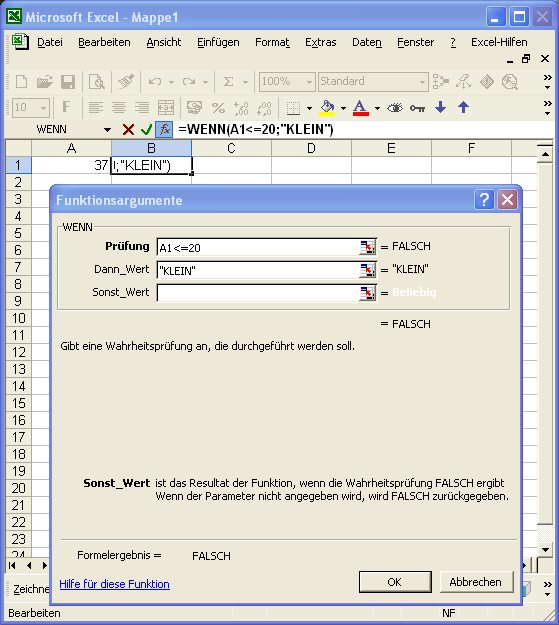Figure 73, dialog for the first IF function

Click in the Else Value field and then call an IF function again. To do this, click on the IF button, which is located immediately above the column header of column A.

The dialog box for entering the function arguments opens again, this time for the second, the nested IF function.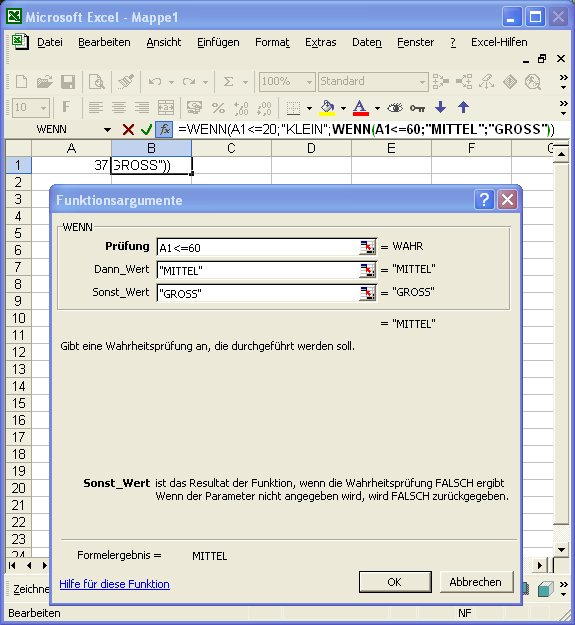Figure 74, dialog for the second IF function

If you now have problems with orientation: Look in the editing line, there you will see the formula that has been started. It contains the IF function twice; the part that you are currently editing in the "Function Arguments" dialog box is shown in bold (see Figure 74).

The editing line is ideal for navigating nested formulas: If you click on a function name, EXCEL will show you the associated dialog box. You can make changes without worrying about the syntax of the notation.

For example, if you click back in the first IF function of the editing line in the current editing state, EXCEL will show you the formula, which has now been completed, in the dialog box according to Figure 75.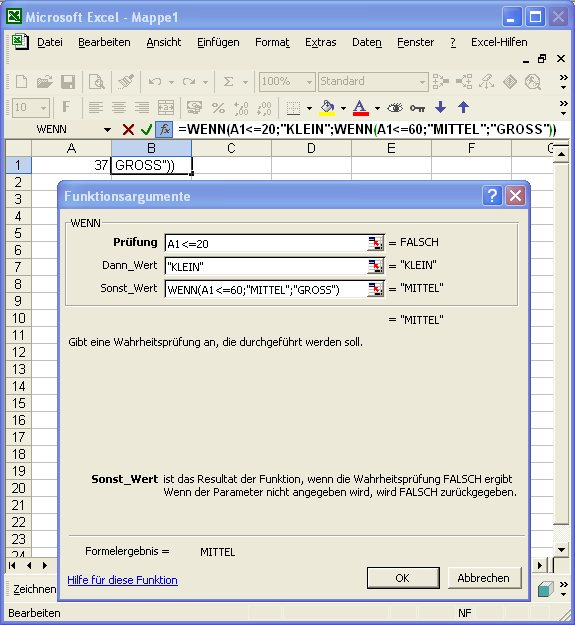Figure 75, The first IF function complete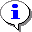If you are working with nested functions, you should always check all functions in the manner just described before entering the formula: Make sure that all arguments are complete!

Didn't find what you're looking for? Enter your search term here!More: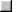Exercises on the IF function